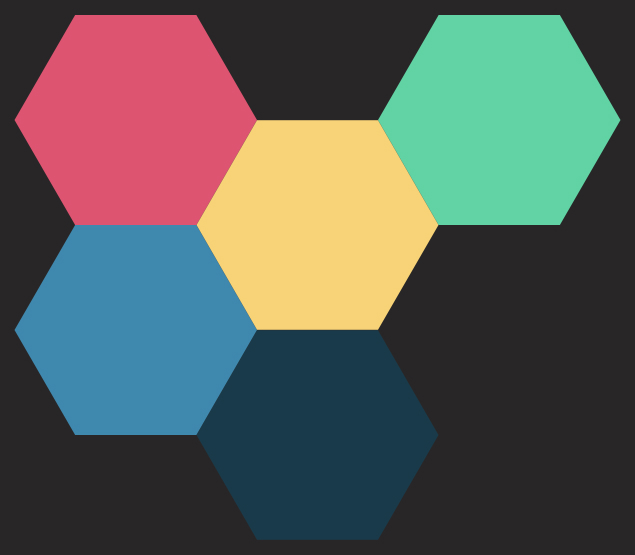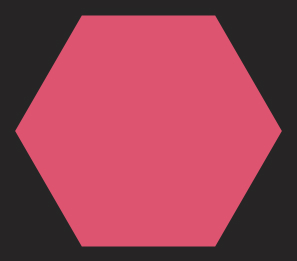Articles about Swift development, by Konstantin Semianov

# Building a hexagonal grid with the SwiftUI Layout protocolThe component we are about to make is available as a Swift Package.

## Intro

SwiftUI is really good at building a hierarchy of rectangular frames. With the recent addition of `Grid` it became even better. However, today we want to build a crazy hexagonal layout. Of course, there is no dedicated layout type for this. So we build our own with the `Layout` protocol!

## Drawing one hexagon

Let's first define a shape for our grid cell. For this, we need to implement `func path(in rect: CGRect) -> Path` to satisfy `Shape` protocol requirement. We basically need to find the largest size of a hexagon that fits inside the rect, compute its vertices and draw lines between them. Here is the complete code to do a flat-top hexagon.

``````struct Hexagon: Shape {
static let aspectRatio: CGFloat = 2 / sqrt(3)

func path(in rect: CGRect) -> Path {
var path = Path()

let center = CGPoint(x: rect.midX, y: rect.midY)
let width = min(rect.width, rect.height * Self.aspectRatio)
let size = width / 2
let corners = (0..<6)
.map {
let angle = -CGFloat.pi / 3 * CGFloat(\$0)
let dx = size * cos(angle)
let dy = size * sin(angle)

return CGPoint(x: center.x + dx, y: center.y + dy)
}

path.move(to: corners)
corners[1..<6].forEach { point in
}

path.closeSubpath()

return path
}
}
``````## Coordinates

We'll need to place our hexagons somewhere. And for that, we need a coordinate system. The easiest to understand is the offset coordinate system, but other coordinates could be used with the same success (e.g. axial coordinates). We'll take an odd-q variation of the offset coordinates. It basically just defines cells as pairs of rows and columns. And each odd column is shifted by 1/2 down. We will need to provide these coordinates to the layout system and it's done by creating a key conforming to `LayoutValueKey`.

``````struct OffsetCoordinate: Hashable {
var row: Int
var col: Int
}

protocol OffsetCoordinateProviding {
var offsetCoordinate: OffsetCoordinate { get }
}

struct OffsetCoordinateLayoutValueKey: LayoutValueKey {
static let defaultValue: OffsetCoordinate? = nil
}
``````

## Layout protocol

The protocol has 2 requirements:

• `sizeThatFits` controls how much space the view needs
• `placeSubviews` controls the placement of subviews within the available space

And optionally:

• `makeCache` to avoid extra computations

## Caching

Let's define our cached data for the layout protocol. First, we'll need to know the top left coordinates of the grid to correctly calculate offsets from the bounds' top left corner. Then we'll need to know how big is the grid in terms of full rows and columns of cells.

``````struct CacheData {
let offsetX: Int
let offsetY: Int
let width: CGFloat
let height: CGFloat
}

func makeCache(subviews: Subviews) -> CacheData? {
let coordinates = subviews.compactMap { \$0[OffsetCoordinateLayoutValueKey.self] }

if coordinates.isEmpty { return nil }

let offsetX = coordinates.map { \$0.col }.min()!
let offsetY = coordinates.map { \$0.row }.min()!

let coordinatesX = coordinates.map { CGFloat(\$0.col) }
let minX: CGFloat = coordinatesX.min()!
let maxX: CGFloat = coordinatesX.max()!
let width = maxX - minX + 4 / 3

let coordinatesY = coordinates.map { CGFloat(\$0.row) + 1 / 2 * CGFloat(\$0.col & 1) }
let minY: CGFloat = coordinatesY.min()!
let maxY: CGFloat = coordinatesY.max()!
let height = maxY - minY + 1

return CacheData(offsetX: offsetX, offsetY: offsetY, width: width, height: height)
}
``````

## `sizeThatFits`

This one is pretty straightforward. We just need to take the width of the hex cell such that it fits inside the proposal. And then multiply it by the corresponding width and height of the grid in terms of cell width.

``````func sizeThatFits(proposal: ProposedViewSize, subviews: Subviews, cache: inout CacheData?) -> CGSize {
guard let cache else { return .zero }

let size = proposal.replacingUnspecifiedDimensions()
let step = min(size.width / cache.width, size.height / cache.height / Hexagon.aspectRatio)

return CGSize(width: step * cache.width, height: step * cache.height * Hexagon.aspectRatio)
}
``````

## `placeSubviews`

Here we compute the step between subsequent hexagons. And then placing each hexagon at its corresponding place with the correct size.

``````func placeSubviews(in bounds: CGRect, proposal: ProposedViewSize, subviews: Subviews, cache: inout CacheData?) {
guard let cache else { return }

let size = proposal.replacingUnspecifiedDimensions()
let step = min(size.width / cache.width, size.height / cache.height / Hexagon.aspectRatio)
let width = step * 4 / 3
let proposal = ProposedViewSize(width: width, height: width / Hexagon.aspectRatio)
let x = width / 2 + bounds.minX
let y = width / Hexagon.aspectRatio / 2 + bounds.minY

for subview in subviews {
guard let coord = subview[OffsetCoordinateLayoutValueKey.self] else { continue }

let dx: CGFloat = step * CGFloat(coord.col - cache.offsetX)
let dy: CGFloat = step * Hexagon.aspectRatio * (CGFloat(coord.row - cache.offsetY) + 1 / 2 * CGFloat(coord.col & 1))
let point = CGPoint(x: x + dx, y: y + dy)

subview.place(at: point, anchor: .center, proposal: proposal)
}
}
``````

## HexGrid

At this point, the `HexLayout` is already usable. However, the rule that all subviews should have a coordinate is not enforced. So it's better to do a thin wrapper that will provide this compile-time guarantee to component consumers. While at it, we'll clip the subviews with the shape of the hexagon to make the call site even cleaner.

``````struct HexGrid<Data, ID, Content>: View where Data: RandomAccessCollection, Data.Element: OffsetCoordinateProviding, ID: Hashable, Content: View {
let data: Data
let id: KeyPath<Data.Element, ID>
let content: (Data.Element) -> Content

init(_ data: Data,
id: KeyPath<Data.Element, ID>,
@ViewBuilder content: @escaping (Data.Element) -> Content) {
self.data = data
self.id = id
self.content = content
}

var body: some View {
HexLayout {
ForEach(data, id: id) { element in
content(element)
.clipShape(Hexagon())
.layoutValue(key: OffsetCoordinateLayoutValueKey.self,
value: element.offsetCoordinate)
}
}
}
}
``````
``````extension HexGrid where ID == Data.Element.ID, Data.Element: Identifiable {
init(_ data: Data,
@ViewBuilder content: @escaping (Data.Element) -> Content) {
self.init(data, id: \.id, content: content)
}
}
``````

## Usage

Now we can finally define our data model and use the ready component to get the image from the beginning of the article:

``````struct HexCell: Identifiable, OffsetCoordinateProviding {
var id: Int { offsetCoordinate.hashValue }
var offsetCoordinate: OffsetCoordinate
var colorName: String
}

let cells: [HexCell] = [
.init(offsetCoordinate: .init(row: 0, col: 0), colorName: "color1"),
.init(offsetCoordinate: .init(row: 0, col: 1), colorName: "color2"),
.init(offsetCoordinate: .init(row: 0, col: 2), colorName: "color3"),
.init(offsetCoordinate: .init(row: 1, col: 0), colorName: "color4"),
.init(offsetCoordinate: .init(row: 1, col: 1), colorName: "color5")
]

HexGrid(cells) { cell in
Color(cell.colorName)
}
``````

But you can put images or literally any view into subviews! Just be aware that the layout assumes subviews fill the contents of the hexagon cell.

``````HexGrid(cells) { cell in
AsyncImage(url: cell.url) { image in
image.resizable().aspectRatio(contentMode: .fill)
} placeholder: {
ProgressView().frame(maxWidth: .infinity, maxHeight: .infinity)
}
}
``````
We've learned how to provide values to `LayoutSubview` proxy and build a fun non-trivial layout.